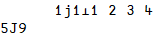# Fast.ai APL study session 14

Discussion of the 14th study group can go here. Overview

This is a wiki post - feel free to edit to add links from the lesson or other useful info.

## Study session resources

1 Like

I can’t find extensions to `⍱` and `⍲` due to the existing extensions to `∨` and `∧`.

GCD and LCM are just one of the possible extensions to OR and AND. Another would have been Max and Min:

``````      (0 1 0 1 ∨ 0 0 1 1) ≡ (0 1 0 1 ⌈ 0 0 1 1)
1
(0 1 0 1 ∧ 0 0 1 1) ≡ (0 1 0 1 ⌊ 0 0 1 1)
1
``````

But this wouldn’t have led to any extensions for NOR and NAND either. A third possibility would have been extending AND to Times and NOT to subtraction from 1:

``````      (0 1 0 1 ∧ 0 0 1 1) ≡ (0 1 0 1 × 0 0 1 1)
1
(~ 0 1 0 1) ≡ (1 - 0 1 0 1)
1
``````

Then, with De Morgan’s laws, we could have defined all other logical functions:

``````      (0 1 0 1 ⍲ 0 0 1 1) ≡ (1 - 0 1 0 1 × 0 0 1 1)
1
(0 1 0 1 ⍱ 0 0 1 1) ≡ ((1-0 1 0 1) × (1-0 0 1 1))
1
(0 1 0 1 ∨ 0 0 1 1) ≡ (1 - (1-0 1 0 1) × (1-0 0 1 1))
1
``````

What would this represent? Probability. E.g. If I have a 25% chance of going out AND there’s a 50% chance of rain, what is the chance I’m getting wet?

``````      AND ← ×
0.25 AND 0.50
0.125
``````

There’s also a 40% chance I’ll take a shower. What’s the chance I’ll get wet from showering OR rain?

``````      NOT←1∘-
OR←{NOT (NOT ⍺) AND (NOT ⍵)}
0.40 OR 0.25 AND 0.50
0.475
``````

And the chance I’ll stay dry, i.e. I’ll neither shower NOR experience rain?

``````      NOR←NOT OR
0.40 NOR 0.25 AND 0.50
0.525
``````

Back to reality: Earlier APLers choose GCD and LCM over multiplicative fuzzy logic, and so `⍱` `⍲` `~` will likely remain with their limited domain. Also, sometimes people use `~~` to assert a Boolean argument, causing a DOMAIN ERROR on anything else.

1 Like

You need mnemonics so you don’t have to keep asking for the keyboard locations of glyphs. E.g. Enclose (`⊂`) zips up its argument into a scalar form and Pick (`⊃`) extracts elements from an array, while L looks like the lower left half of `⎕`.

4 Likes

### `a/⍨≠a` as a train

There’s a problem of `/` preferring to be an operator instead of a function, so we have to convince it to be a function, e.g. by making it an operand to an operator (because functions can be operands, but operators cannot). We can use the atop operator `⍤` but since we don’t actually want any post-processing, the left operand will be the identity function `⊢`. We can thus write the function `/` as `⊢⍤/`.

With that sorted, we want the left argument to be the result of `≠` and the right argument to be the result of… well, we just want the argument, so `⊢` again:

``````      ∪2 3 4 2 2 3 4 5
2 3 4 5
a/⍨≠a←2 3 4 2 2 3 4 5
2 3 4 5
(≠⊢⍤/⊢)2 3 4 2 2 3 4 5
2 3 4 5
``````

But maybe a little dfn would be better here:

``````      {⍵/⍨≠⍵}2 3 4 2 2 3 4 5
2 3 4 5
``````

I’m actually proposing an additional composition operator `f⍛g Y` which would mean `(f Y) g Y` (denoted `b` here, for behind):

``````      b←{(⍺⍺ ⍵)⍵⍵ ⍵}
≠b/2 3 4 2 2 3 4 5  ⍝ ≠⍛/
2 3 4 5
``````

### `⊤` and `⊥` with higher-rank arguments

Each digit position is represented as a major cell:

``````      ⎕←m←2 2 2 2 ⊤ 3 1 4
0 0 0  ← ×  2×2×2×1
0 0 1  ← ×    2×2×1
1 0 0  ← ×      2×1
1 1 0  ← ×        1

↑ ↑ ↑
3 1 4
``````

3 = (0,0,1,1)2
1 = (0,0,0,1)2
4 = (0,1,0,0)2
Having the numbers be “vertical” may seem surprising, but it is because, if we compute the digit weights…

``````      ⎕←w←⌽×\⌽1↓2 2 2 2,1
8 4 2 1
``````

… we can use dot product/matrix multiplication (which traditional mathematics defines as summing the products of columns from the left with rows from the right) to compute the total values:

``````      w +.× m
3 1 4
``````

Mixed-radix example (100 and 1440 minutes in days,hours,minutes):

``````      ⎕←m←7 24 60 ⊤ 100 1440
0 1  ←  ×  24×60×1
1 0  ←  ×     60×1
40 0  ←  ×        1

↑ ↑
100└1440
⌽×\⌽1↓7 24 60,1
1440 60 1
1440 60 1 +.× m
100 1440
``````

With a matrix left argument, each column represents a radix and we do an outer product-like operation, combining all radices with all numbers:

``````      (3 2⍴10 16)⊤128 256
┌─1 2┐ ←  ×  10×10×1
│┌0 1│┐←  ×  16×16×1
││   ││
├│2 5┤│←  ×     10×1
│├8 0│┤←  ×     16×1
││   ││
└│8 6┘│←  ×        1
└0 0─┘←  ×        1

↑ ↑
128 256
``````

128 = (1,2,8)10
256 = (2,5,6)10
128 = (0,8,0)16
256 = (1,0,0)16
Again here, we have the first major cell (a layer of the rank-3 result) represent all the highest-valued digits, 10×10×1 and 16×16×1 for each of the 100 and 200 parts of 128 and 256.

Regarding 55:20 of the video, I worked out the polynomial evaluation for the complex number example. This makes me appreciate APL more.1 Like